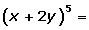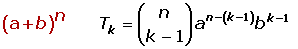Chapters

The following formula allows one to find the powers of a binomial. It is known as the binomial theorem.Observe that:

The number of terms is n + 1.

The coefficients are combinatorial numbers corresponding to the nth row of Pascal's triangle.In the development of the binomial, the exponents of a are decreasing, one by one, from n to zero; and the exponents of b are increasing, one by one, from zero to n, therefore, the sum of the exponents of a and b in each term is equal to n.

In the case that one of the terms of the binomial is negative, alternate the positive and negative signs.

## Examples

1.2.3.4.5.6.### Calculation of the Term which Occupies the Place k## Examples

1. Find the fifth term of the development.2.Find the fourth term of the developmentis:3.Find the eighth term of the development4.Find the fifth term of the development.5.Find the independent term of the development.The exponent of a with the independent term is 0, therefore, take only the literal part and equal it to a0.Did you like the article?(No Ratings Yet)Loading...

Emma

I am passionate about travelling and currently live and work in Paris. I like to spend my time reading, gardening, running, learning languages and exploring new places.

Did you like
this resource?

Bravo!